Match Fishtank is now Fishtank Learning!

# Transformations and Angle Relationships

## Objective

Describe and perform reflections between congruent figures. Use reflections to determine if figures are congruent.

## Common Core Standards

### Core Standards

?

• 8.G.A.1.A — Lines are taken to lines, and line segments to line segments of the same length.

• 8.G.A.1.B — Angles are taken to angles of the same measure.

• 8.G.A.1.C — Parallel lines are taken to parallel lines.

• 8.G.A.2 — Understand that a two-dimensional figure is congruent to another if the second can be obtained from the first by a sequence of rotations, reflections, and translations; given two congruent figures, describe a sequence that exhibits the congruence between them.

## Criteria for Success

?

1. Use patty (transparency) paper to reflect images over horizontal and vertical lines.
2. Describe a reflection using a line of reflection.
3. Understand that every point on an original image is equidistant from the line of reflection as each corresponding point on the reflected image.
4. Understand that reflections preserve shape, size, area, perimeter, and parallel line relationships, but they do not preserve orientation or location.

## Tips for Teachers

?

• Lessons 4 and 5 are on reflections. Lesson 4 focuses on students understanding how to perform reflections and how to talk about them. Students use patty paper to reinforce concepts through experimentation and verification (MP.5). In Lesson 5, students start to work with combinations of transformations, combining reflections with translations learned in previous lessons.
• This video, Reflection, by PBS Learning Media introduces the concept of reflection and includes animation that can be helpful for students to visualize the movements
• The following materials are useful for this lesson: patty paper (or transparency paper) and graph paper.

#### Remote Learning Guidance

If you need to adapt or shorten this lesson for remote learning, we suggest prioritizing Anchor Problem 1 (benefits from discussion) and Anchor Problem 2 (benefits from worked example). Find more guidance on adapting our math curriculum for remote learning here.

#### Fishtank Plus

• Problem Set
• Student Handout Editor
• Vocabulary Package

## Anchor Problems

?

### Problem 1

Figure ${ABCDEF}$ is shown in the coordinate plane below. Trace the figure on a piece of patty paper, then use it to investigate the questions that follow.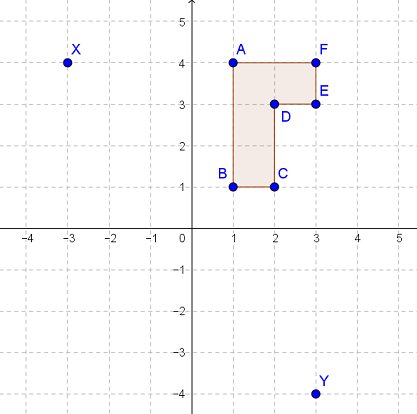1. Use your patty paper to reflect the figure so that point $F$ maps to point $X$. Draw the reflected image in the coordinate plane. How would you describe the reflection?
2. Use your patty paper to reflect the original figure so that point $F$ maps to point $Y$. Draw the reflected image in the coordinate plane. How would you describe the reflection?
3. What impact does reflecting an image have on its orientation? How is this different from a translation?

### Problem 2

Triangle ${EFG}$ is reflected across a line of reflection to map to triangle ${E'F'G'}$ as shown below.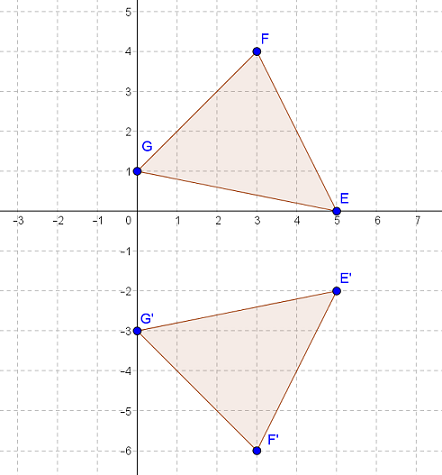What is the line of reflection?

## Problem Set

?The following resources include problems and activities aligned to the objective of the lesson that can be used to create your own problem set.

• Examples where students perform reflections given a figure and a line of reflection (include x- and y-axes as well as other horizontal and vertical lines)
• Examples where students are given two figures (some reflected and some not correctly reflected) and determine if they are congruent; name the line of reflection or explain the error in reflection

?

In each diagram below, determine if Figure 2 can be obtained by a single reflection of Figure 1. If yes, then describe the reflection.

a.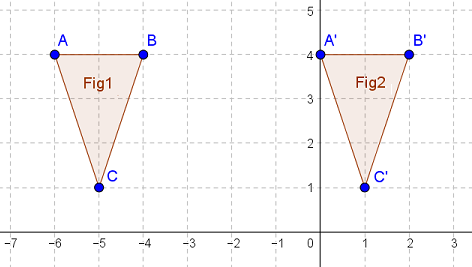b.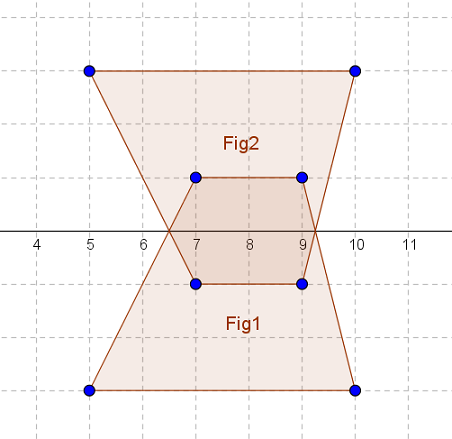c.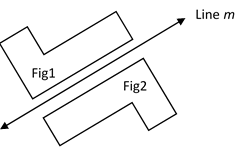?